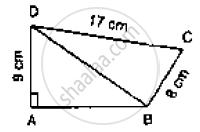# In the Below Figure, Compute the Area of Quadrilateral Abcd. - Mathematics

Sum

In the below figure, compute the area of quadrilateral ABCD.#### Solution

Given that
DC = 17cm

AD = 9cm and BC = 8cm

In ΔBCD we have

CD2 = BD 2 + BC2

⇒  (17)2 = BD2 + (8)2

⇒ BD2 = 289 - 64

⇒ BD  = 15

In ΔABD, we have

⇒ (15)2  = AB2  + (9)2

⇒ AB2  = 225 - 81 = 144

⇒  AB = 12

ar ( quad , ABCD) =ar ( ΔABD) + ar ( Δ BCD)

⇒ ar ( quad , ABCD) = 1/2 ( 12 × 9 ) + 1/2 (8 × 17 ) = 54 + 68

= 112cm2

⇒ ar (quad, , ABCD =1/2(12 × 9 ) +1/2 ( 8  ×  15 )

= 54 + 60cm2

= 114cm2

Concept: Corollary: A rectangle and a parallelogram on the same base and between the same parallels are equal in area.
Is there an error in this question or solution?

#### APPEARS IN

RD Sharma Mathematics for Class 9
Chapter 14 Areas of Parallelograms and Triangles
Exercise 14.3 | Q 1 | Page 44

Share